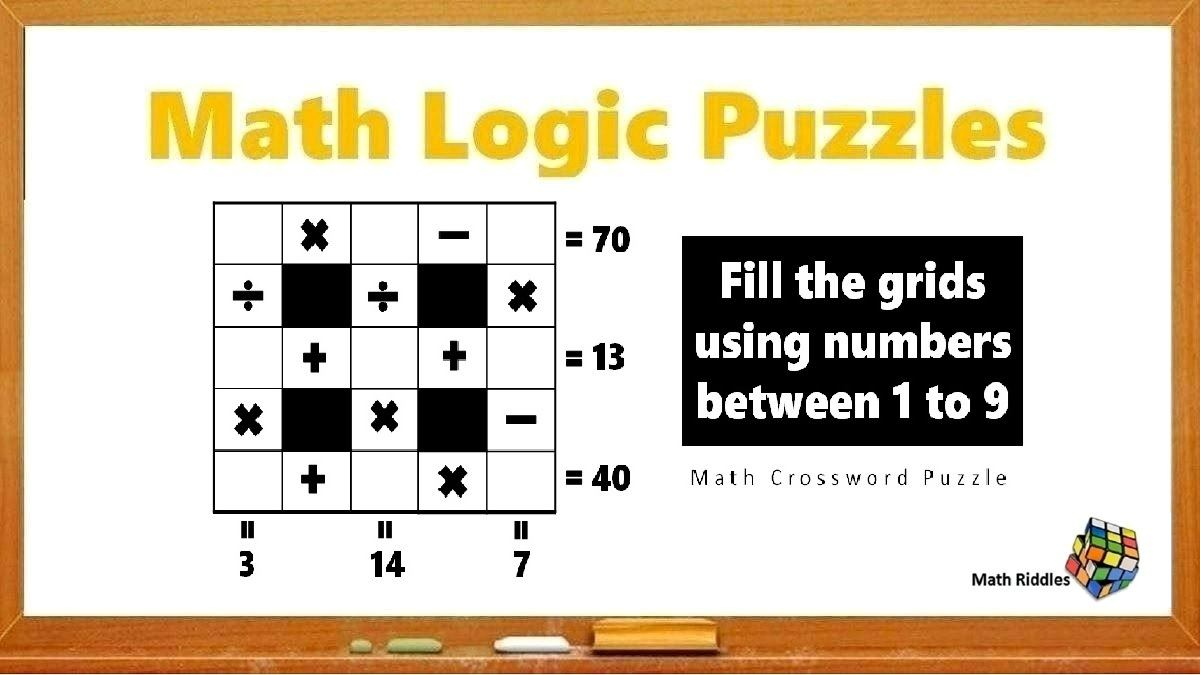# Math Riddles: Solve This Math Crossword Puzzle, Only with 1 to 9 Numbers

Math Riddles Challenge: Using only numbers between 1 to 9, fill the empty puzzle boxes with the missing numbers in less than a 1 minute.Math Riddles: Solve This Math Crossword Puzzle, Using Only with 1 to 9 Numbers

Math Riddles: Today, we have a freshly pressed puzzle in the series of our Jagran Josh Math Riddles. In this article, we have a Math Crossword Puzzle where you have to fill up the empty squares of the puzzle using numbers between 1 to 9 only. Mind you, all numbers from 1 to 9 have to be used without repetition in a way that they satisfy the equations in the puzzle. Let's do some brain exercises.

## Solution

Math Crossword Puzzles With Answers #1

Explanation:

Before we start solving, we have to keep in mind that calculations are done from left to right and top to bottom. Let us begin solving:

Taking up the first row of the crossword puzzle, we figure the missing numbers between 1 to 9 that when multiplied and subtracted gives us 70. So, we try all multiplication combinations while skipping repetitive products. While doing so, we get 8 x 9 = 72.

Using logic, we can figure that if we subtract 2 from 72, we shall get 70. So, here we may have figured out the possible numbers in the top row.

However, is it 8 x 9 – 2 or 9 x 8 – 2 can only be figured after we solve the first column top to bottom.

Here, we have two possibilities figured out in the top left empty square which could either be an 8 or 9. So, we try different combinations and see that only 9 divided by 3 multiplied by 1 can give us 3 as in the puzzle.

Now, our math crossword puzzle looks like this:

Now, moving forward, we got four empty squares and four numbers left (4, 5, 6, 7).

Now, we solve the second column wherein we already have an 8 vertically and a 3 horizontally. So, we try several combinations and figure that 8 divided by 4 multiplied by 7 will get us 14 as in the puzzle.

Now, our math crossword puzzle looks like this:

Now, we solve the third column which should be easy as we have figured out more than 80 per cent of the puzzle, We got two numbers left now: 5 and 6. So, we have already figured a 2 vertically. So, 2 multiplied by 6 minus 5 will get us 7 as in the puzzle. Also, we see that all numbers satisfy the equations horizontally as well.

Horizontal Equations:

=> 9 x 8 – 2 = 70

=> 3 + 4 + 6 = 13

=> 1 + 7 x 5 = 40

Vertical Equations

=> 9  3 x 1 = 3

=> 8  4 x 7 = 14

=> 2 x 6 – 5 = 7

Now, our math crossword puzzle looks like this:

Math Crossword Solved!

## Tell us in comments: Did you solve these math puzzles in 20 seconds each?

Check out more math puzzles!

Also Read: Math Riddles: Find Missing Shapes Quiz, Can You Solve in 20 Seconds Each?

Get the latest General Knowledge and Current Affairs from all over India and world for all competitive exams.
खेलें हर किस्म के रोमांच से भरपूर गेम्स सिर्फ़ जागरण प्ले पर